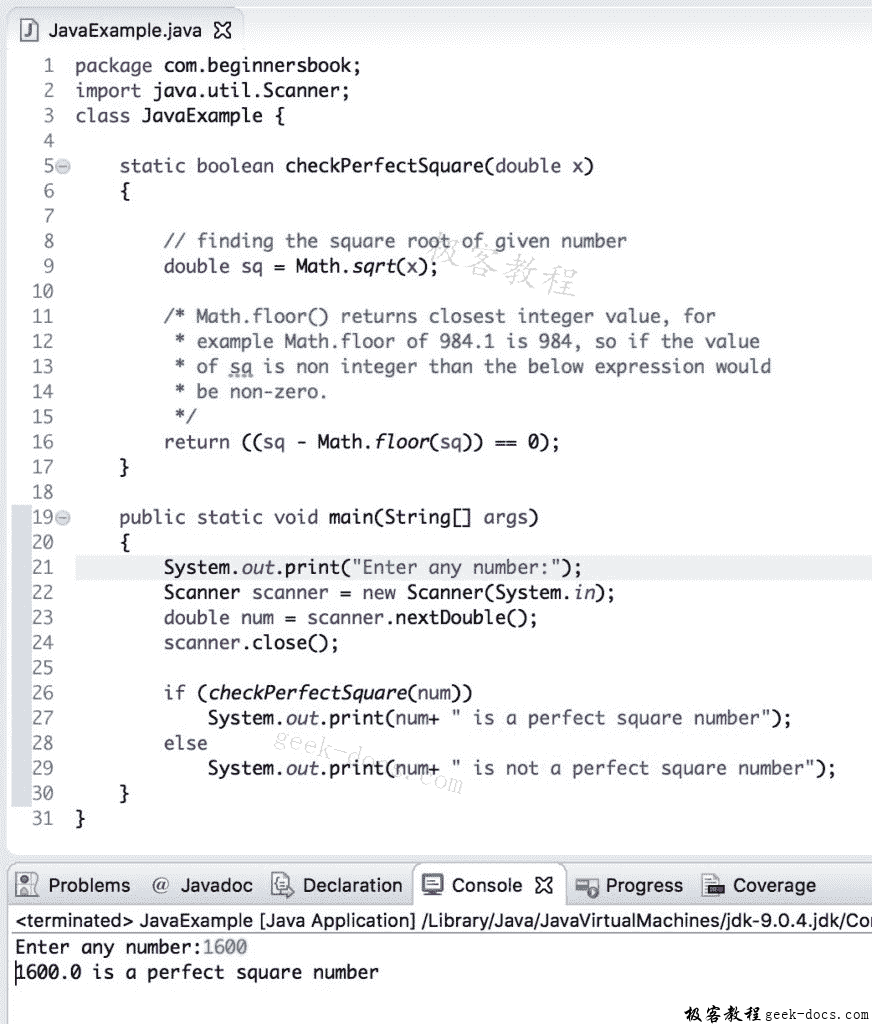# Java 实例 检查给定数字是否为完美平方

Java 示例：检查数字是否是完美平方

package com.beginnersbook;
import java.util.Scanner;
class JavaExample {

static boolean checkPerfectSquare(double x)
{

// finding the square root of given number
double sq = Math.sqrt(x);

/* Math.floor() returns closest integer value, for
* example Math.floor of 984.1 is 984, so if the value
* of sq is non integer than the below expression would
* be non-zero.
*/
return ((sq - Math.floor(sq)) == 0);
}

public static void main(String[] args)
{
System.out.print("Enter any number:");
Scanner scanner = new Scanner(System.in);
double num = scanner.nextDouble();
scanner.close();

if (checkPerfectSquare(num))
System.out.print(num+ " is a perfect square number");
else
System.out.print(num+ " is not a perfect square number");
}
}### 评论 1

1. #1

我实在忍不住想问。可以将解决思路或逻辑先写出来吗？而不是说怎样写代码。很多是不知道检查数字是否是完美正方形的数学逻辑，并非是如何写代码。

mianmian1年前 (2020-03-06)回复
• 回顶